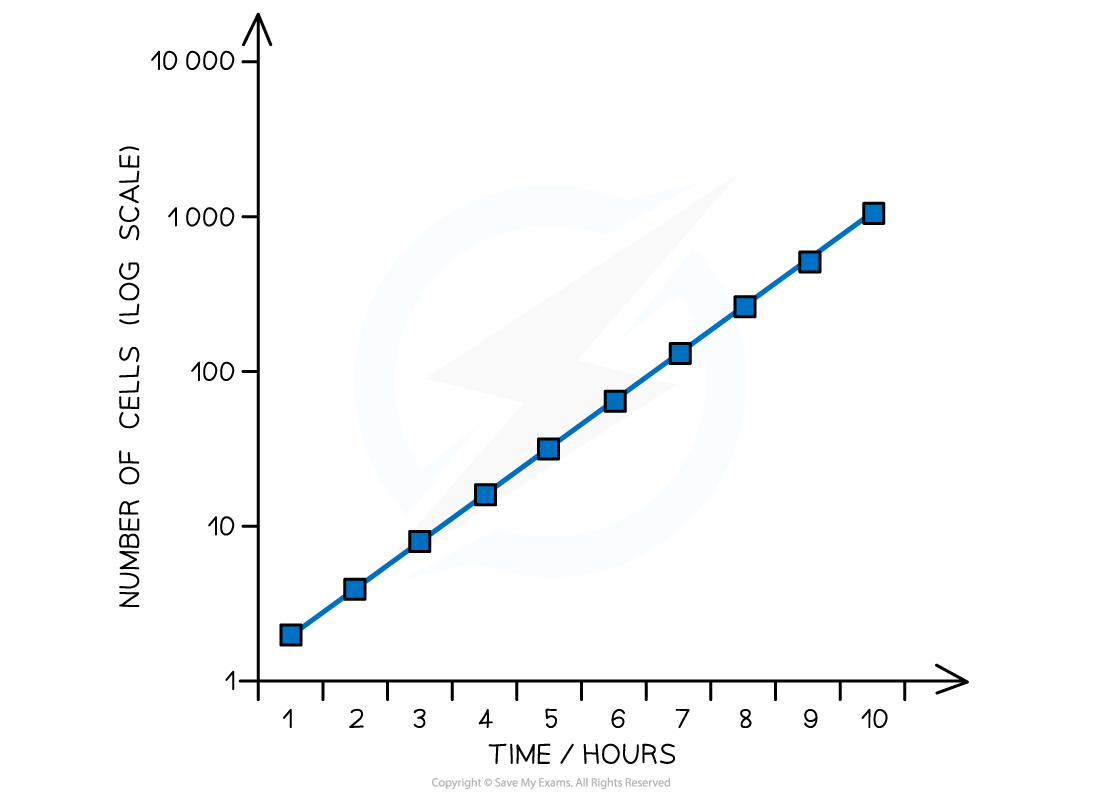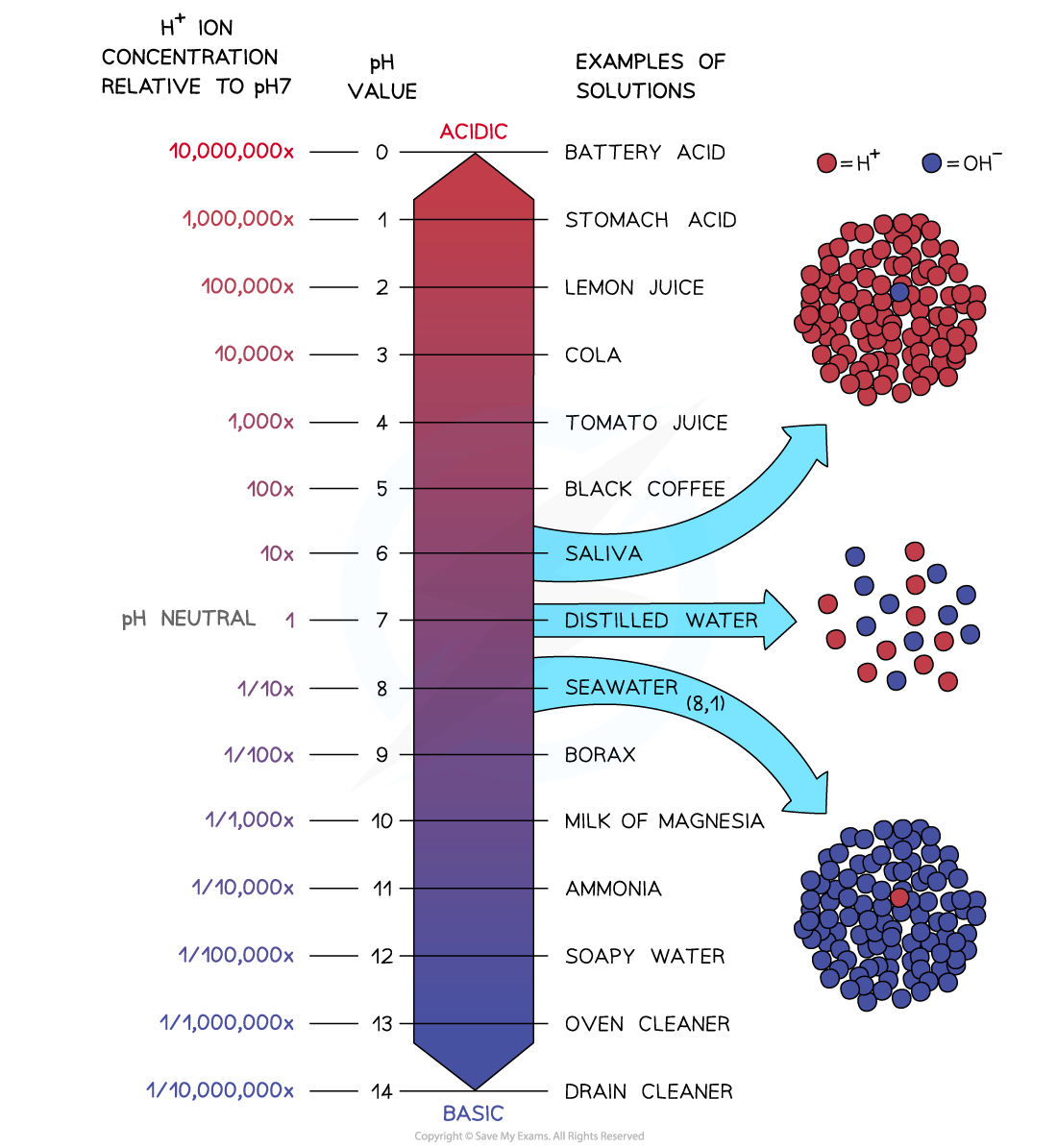# AQA A Level Biology复习笔记4.4.2 Maths Skill: Using Logarithms When Investigating Bacteria

### Maths Skill: Using Logarithms When Investigating Bacteria

• Bacterial colonies can grow at rapid rates when in culture with very large numbers of bacteria produced within hours
• Dealing with the experimental data relating to large numbers of bacteria can be difficult when using traditional linear scales
• There is a wide range of very small and very large numbers
• This makes it hard to work out a suitable scale for the axes of graphs

• Logarithmic scales can be very useful when investigating bacteria

Using logarithms to deal with orders of magnitude

• Logarithmic scales allow for a wide range of values to be displayed on a single graph
• For example, yeast cells were grown in culture over several hours. The number of cells increased very rapidly from the original number of cells present
• The results from the experiment are shown in the graph below, using a log scale
• The number of yeast cells present at each time interval was converted to a logarithm before being plotted on the graph
• The log scale is easily identifiable as there are not equal intervals between the numbers on the y-axis
• The wide range of cell numbers fit easily onto the same scaleImage showing the number of yeast cells grown in culture over 10 hours, using a logarithmic scale

• The pH scale is logarithmic
• The concentration of hydrogen ions varies massively between each pH levelImage showing the range of hydrogen ion concentrations within the pH scale

#### Exam Tip

You won’t be expected to convert values into logarithms or create a log scale graph in the exam. Instead you might be asked to interpret results that use logarithmic scales or explain the benefit of using one! Remember that graphs with a logarithmic scale have uneven intervals between values on one or more axes.